## BARNYARD [DAY 2]

### Subtraction: Counting Back and Adding Up

Investigate early subtraction strategies through counting back and adding up to find the difference.

## Intentionality & Unit Overview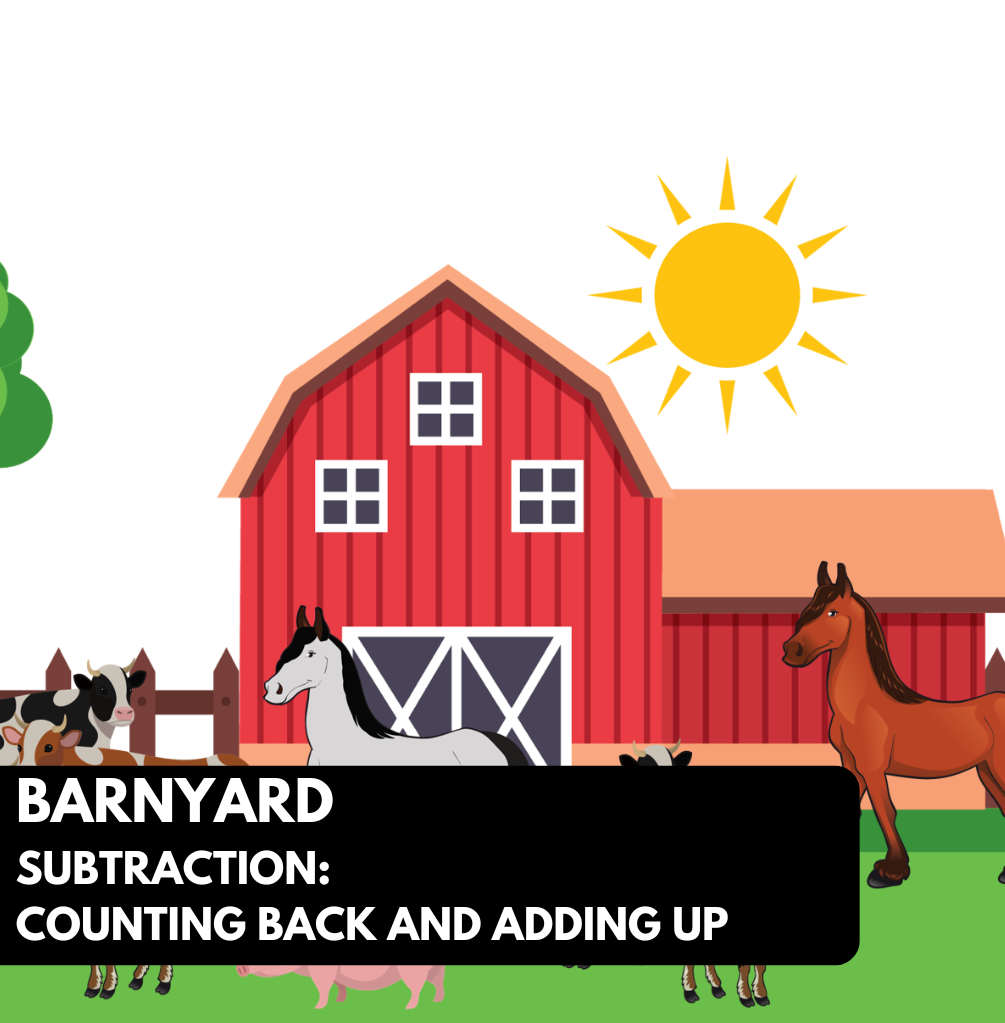### Access each lesson from this unit using the navigation links below

Students will continue exploring early subtraction strategies such as counting back and adding up in a math talk and purposeful practice and small group activities.

The purpose of the Day 2 activities is to reinforce key concepts from Day 1. Students will engage in a math talk and will have an opportunity to complete purposeful practice. The math talk and purposeful practice serve to develop a deeper understanding of the following big ideas.

• Subtraction names the missing part in terms of the whole
• Counting back is efficient when the count back is small
• Addition and subtraction are related in that addition names the parts in the whole and subtraction uses the whole to find a missing part.
• Subtraction can be used in either take away, comparison, or missing addend situations.

## Math Talk

The purpose of today’s math talk is to explore the identity property of subtraction (subtracting 0 from a number does not change the value 4-0=4) and near numbers. It is important for students to recognize how numbers are related to each other. When students are counting, they do not necessarily think about the relationship between numbers, but by recognizing near numbers, it may help students with minus 1 (-1) or minus 2 (-2) facts.

Identity property:

Students need to generalize the idea that when taking away 0 from any number, we are left with the same amount because nothing was taken away. It seems like a concept that could be glossed over but it may be a source of confusion for some students.

## Visual Math Talk Prompt #1

Begin by showing the following silent animation.  After the initial context is set up, be prepared to pause the video where students are prompted with a question to think about.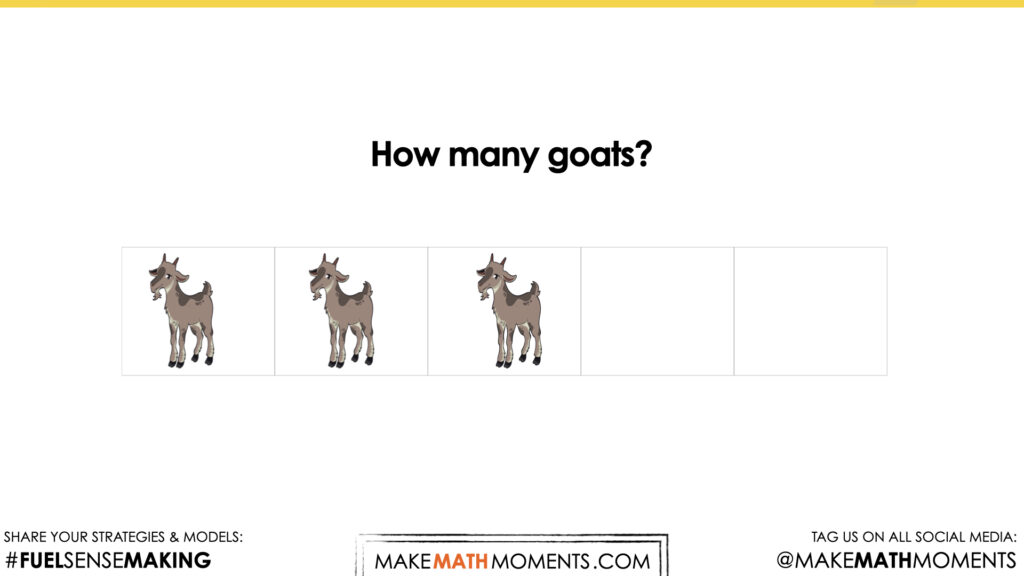This video will pause at this point for students to confirm that there are 3 goats. It is possible that students can subitize this amount. They can “see” that there are 3 goats without having to count them.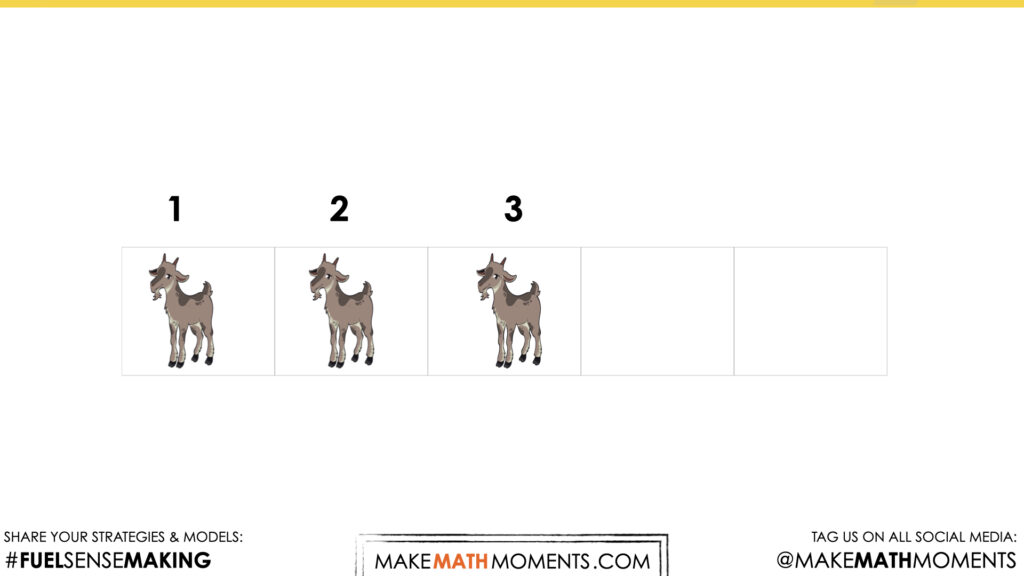This number talk continues by removing one of the goats. As mentioned in Day 1, it is difficult to model a subtraction situation. In this scenario, the subtrahend is being modeled by “removing” the goat from the 5 frame but leaving it under so the subtrahend can be seen/remembered. The video will pause after this prompt to allow students time to process the situation.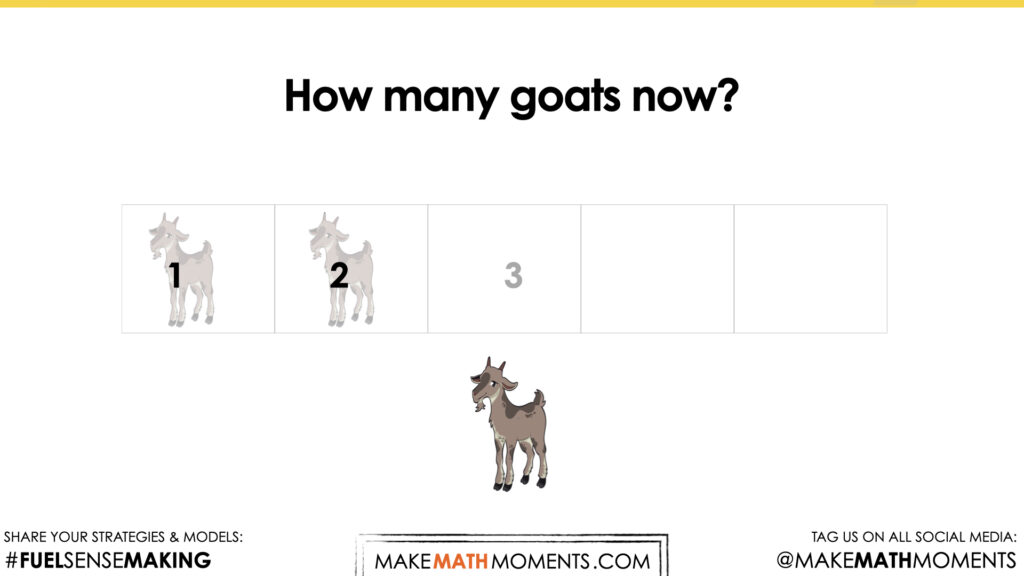To start to emphasize the point of the math talk, consider writing the math sentence that corresponds with this question: 3 – 1 = 2.

Students may count the remaining goats in the five frame, some may be able to subitize the remaining amount and some students may know that 3 minus 1 is 2.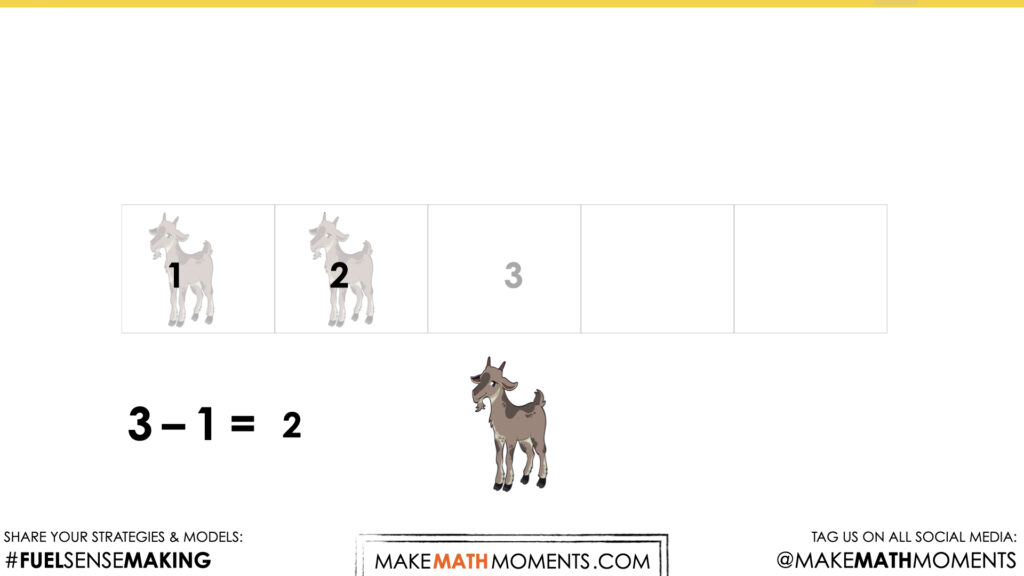## Visual Math Talk Prompt #2

Begin by showing the following silent animation.  After the initial context is set up, be prepared to pause the video where students are prompted with a question to think about.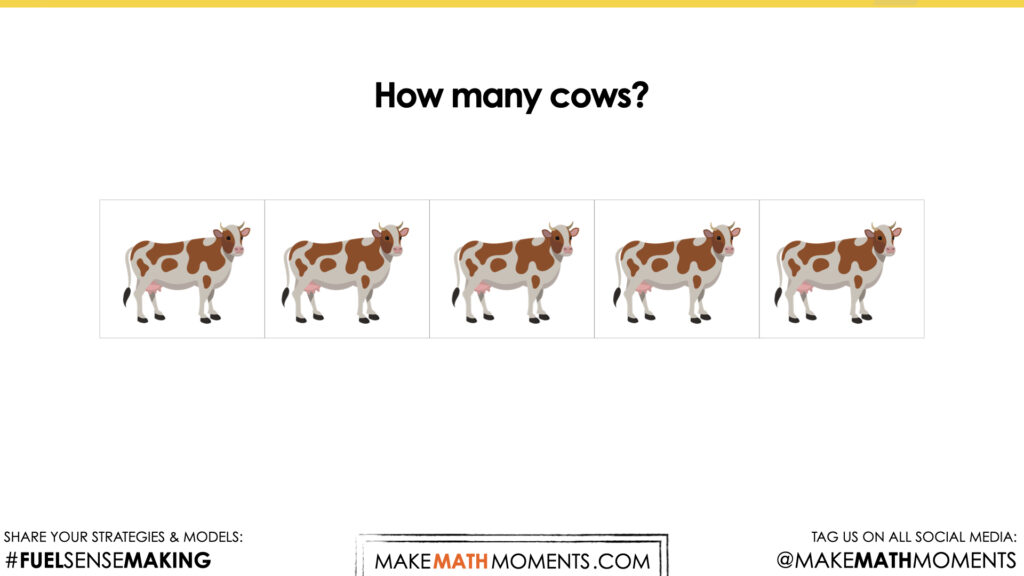A number line is used to represent the 5 cows. This will be later used in the prompt as a visual tool to model the subtraction situation.

This is also an opportunity for students to discuss that a full five frame does not need to be recounted. We can “trust” that there are 5 objects when all 5 spaces are filled.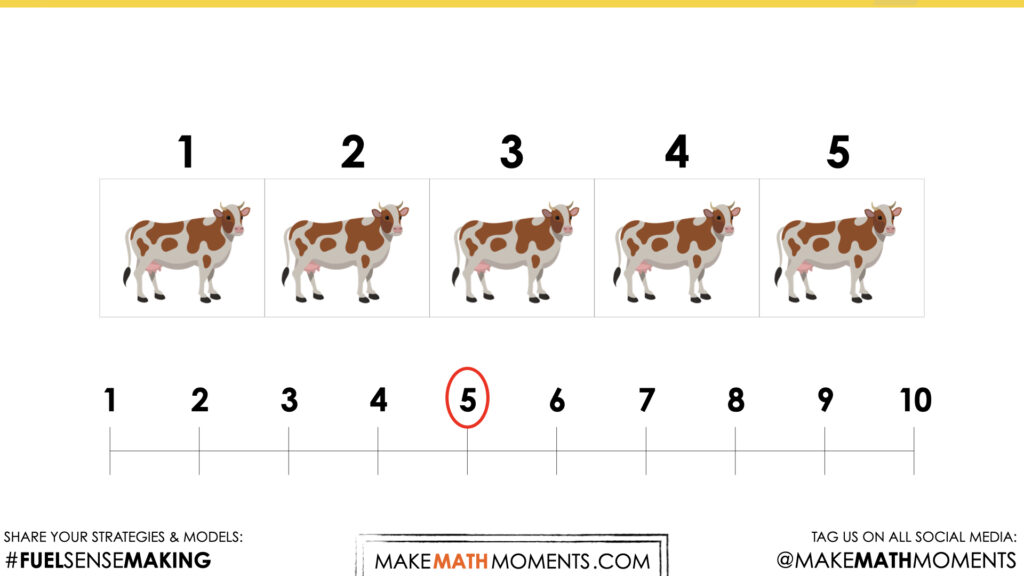Again, the subtraction situation is represented by “removing” one of the cows from the five frame.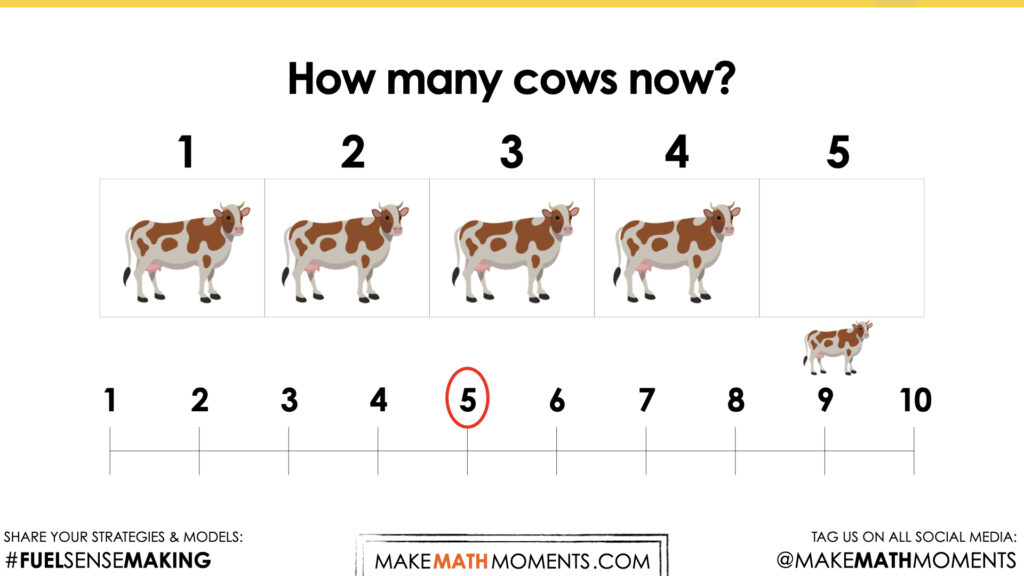As with prompt 1, consider writing the math sentence that corresponds with this question: 5 – 1 = 4.

Students may count the remaining cows in the five frame, some may be able to subitize the remaining amount and some students may know that 5 minus 1 is 4.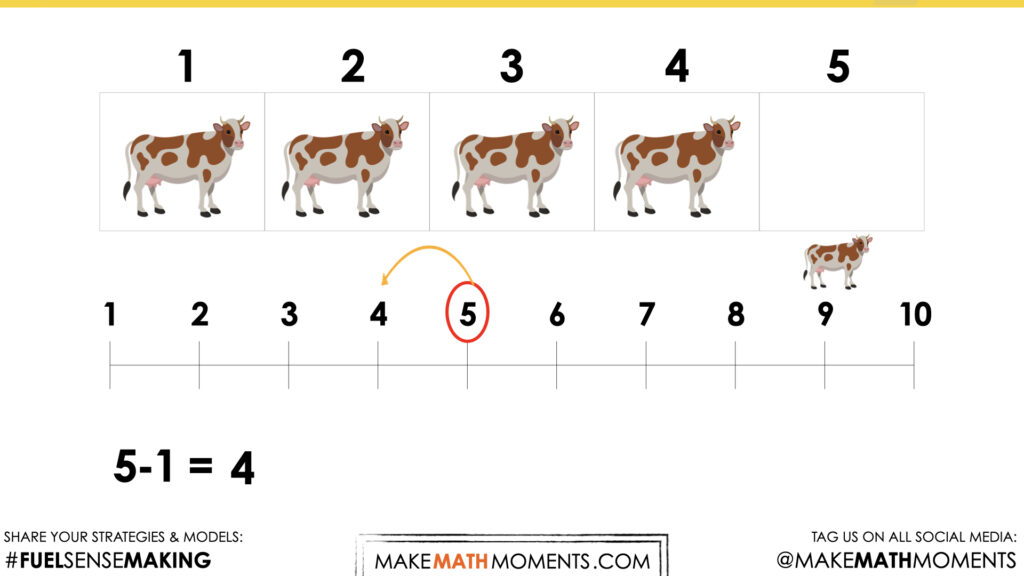## Visual Math Talk Prompt #3

Login/Join to access the entire Visual Prompt, downloadable slide decks and printable handouts for this lesson and all problem based units.

## Visual Math Talk Prompt #4

Login/Join to access the entire Visual Prompt, downloadable slide decks and printable handouts for this lesson and all problem based units.

## Purposeful Practice

Login/Join to access the entire Teacher Guide, downloadable slide decks and printable handouts for this lesson and all problem based units.

## Game: Spinning Back

Login/Join to access the entire Teacher Guide, downloadable slide decks and printable handouts for this lesson and all problem based units.

## Small Group Assessment Opportunity

Login/Join to access the entire Teacher Guide, downloadable slide decks and printable handouts for this lesson and all problem based units.

## Whole Group Activities:

Login/Join to access the entire Teacher Guide, downloadable slide decks and printable handouts for this lesson and all problem based units.

Login/Join to access the entire Teacher Guide, downloadable slide decks and printable handouts for this lesson and all problem based units.

## Educator Discussion Area

Login/Join to access the entire Teacher Guide, downloadable slide decks and printable handouts for this lesson and all problem based units.

## Explore Our 60+ Problem Based Units

This Make Math Moments Lesson was designed to spark curiosity for a multi-day unit of study with built in purposeful practice, number talks and extensions to elicit and emerge strategies and mathematical models.

Dig into our other units of study and view by concept continuum, grade or topic!